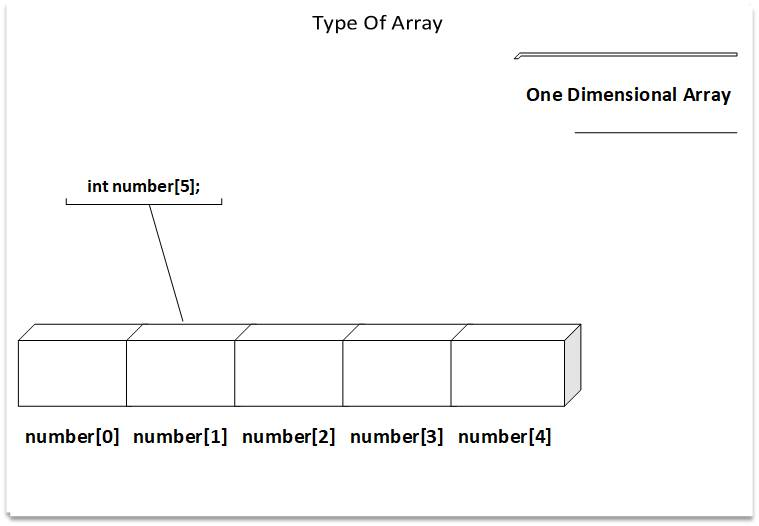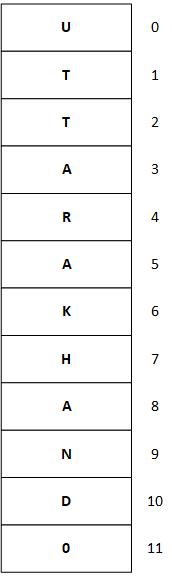# Array C Programing

A list of items can be given one variable name using only one subscript is called a one dimensional arrays.For Example:

If we have to represent five numbers, then we can declare it as:

``````
int number;

``````

On declaring above computer reservers five consecutive memory locations.

``````
number 
number 
number 
number 
number 

``````

We can assign the values to array as:

``````
number =20;
number =25;
number =30;
number =35;
number =40;

``````

C performs no bound checking and, therefore, care should be exercised to ensure that the array indices are within the declared limits.

For Example:

we cannot perform

``````
number  =45;

``````

because it is outside the range of array `number ;`

which can store only 5 elements from `number [O]` to `number `.

One Dimensional Arrays are used to represent all types of lists. These are also used to implement other data structures such as stacks, queues, heaps,etc.

Limitations of One Dimensional Array:

• The prior knowledge of number of elements is necessary
• Arrays are static. Static means, whether memory is allocated at compilation time or run time, their memory cannot be reduced or increased.
• As array elements are stored in consecutive memory locations, the insertion and deletion are time consuming.

Just Like any other variable, arrays must be declared before they are used. The syntax for array declaration is:

``````
type vriable-name[size];

``````

The type specifies the data type of array elements such as int, char, float and size tells the maximum number of elements that can be stored inside the array.

For Example:

``````
int Marks;

``````

delcares the marks to be an array containing 90 whole numbers. Any subscript 0 to 89 are valid.

The C langauge treets, character strings as arrays of character.

For Example:

``````
Char name;

``````

Delcares the name as a character array variable that can hold a maximum of 11 characters. Suppose if we have to store "Uttarakahnd" in it, it would look like this:The null character '\0' shows that termination of string. When declaring character arrays, we must
allow one extra element space for the null character

Initialization of one dimensional Array:

After an array is declared, its elements must be initialized. Otherwise, they will contain "Garbage". We
can initialize the array elements as:

``````
type array-name[size]={ list of values};

``````

The values in the list are seprated by commas.

For Example:

``````
int number  ={0,0,0};

``````

will declare the variable number as an array of size and will assign zero to each element.

If the number of values in the list is less than the number of elements, then only that many elements are initialized. The remaining elements will be set to zero automatically.

For Example:

``````
int number ={1,2,3}

``````

will initialize the first three elements with 1,2,3 and remaining two elements with zero automatically.